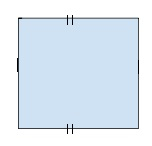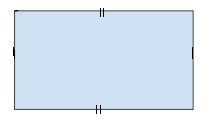# Program to find Perimeter / Circumference of Square and Rectangle in C++

In this problem, we are given the side of a square (A) and the length and breadth of a rectangle (L and B). Our task is to create a Program to find Perimeter / Circumference of Square and Rectangle in C++.

## Problem Description:

• To find the circumference of a square, we need the side of the square (a). For that, we will use the formula for the perimeter of the square which is 4a.

• To find the circumference of a rectangle, we need the Length (L) and breadth (B) of the rectangle. For that, we will use the formula for the perimeter of the rectangle which is 2(L+B).

## Perimeter / Circumference of Square:

Square is a four-sided closed figure that has all four sides equal and all angle 90 degrees.Formula for perimeter / circumference of square = (4 * a)

Let’s take an example to understand the problem,

Input − 5

Output − 20

Input − 12

Output − 48

## Program to illustrate the working of our solution,

#include <iostream>
using namespace std;
int calcCircumference(int a){
int perimeter = (4 * a);
return perimeter;
}
int main() {
int a = 6;
cout<<"The Perimeter / Circumference of Square is
"<<calcCircumference(a);
}

## Output:

The Perimeter / Circumference of Square is 24

## Perimeter / Circumference of Rectangle:

Square is a four-sided closed figure that has opposite sides equal and all angle 90 degrees.Formula for perimeter/circumference of square = 2 * (l + b)

Let’s take an example to understand the problem,

## Example 1:

Input − l = 7; b = 3

Output − 20

## Example 2:

Input − l = 13; b = 6

Output − 38

## Program to illustrate the working of our solution,

#include <iostream>
using namespace std;
int calcCircumference(int l, int b){
int perimeter = (2 * (l + b)); w =
return perimeter;
}
int main() {
int l = 8, b = 5;
cout<<"The Perimeter / Circumference of Rectangle is
"<<calcCircumference(l, b);
}

## Output:

The Perimeter / Circumference of Rectangle is 26

Updated on: 01-Oct-2020

251 Views